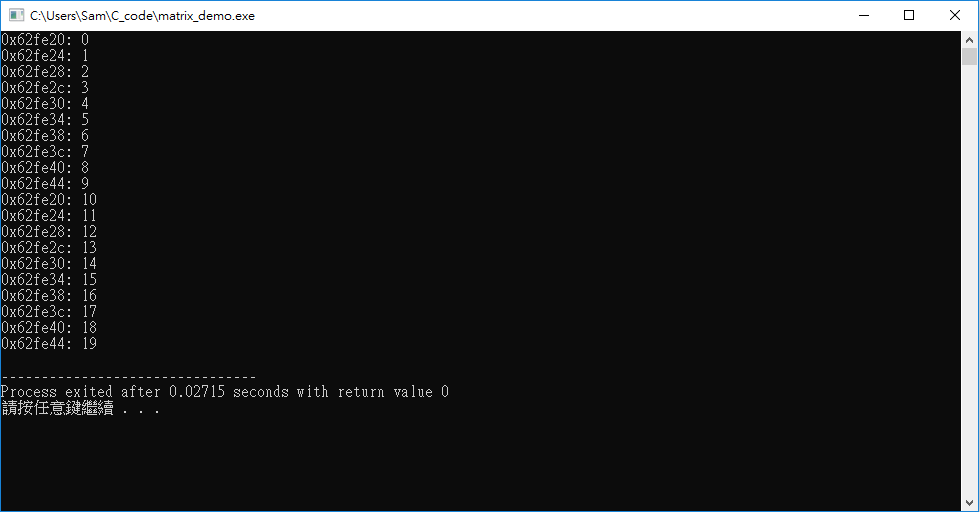#0

## C : 指標與陣列的問題

``````#include <stdio.h>

void matrixChange(int * , int);

int main(void){
int as ={0,1,2,3,4,5,6,7,8,9};

matrixChange(as,10);
return 0;
}

void matrixChange(int *ptr_a , int size){
int count=0;

while(count<size){
printf("%#x: %d\n",ptr_a+count,*ptr_a+count);
count++;
}

count=0;
while(count<size){
*(ptr_a+count) = 10;
printf("%#x: %d\n",ptr_a+count,*ptr_a+count);
count++;
}
}
``````### 1 則留言

2

*(ptr_a+count) = 10; // 指派10給指標的值
printf("%#x: %d\n",ptr_a+count,*ptr_a+count); // *ptr_a取值(10) + count